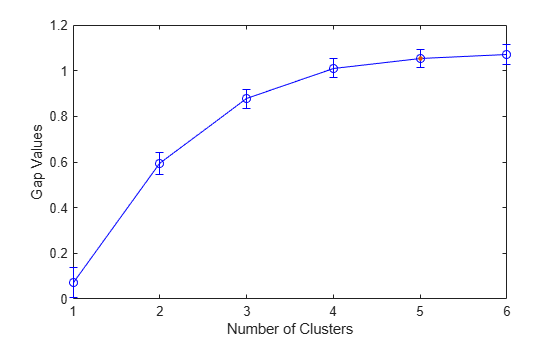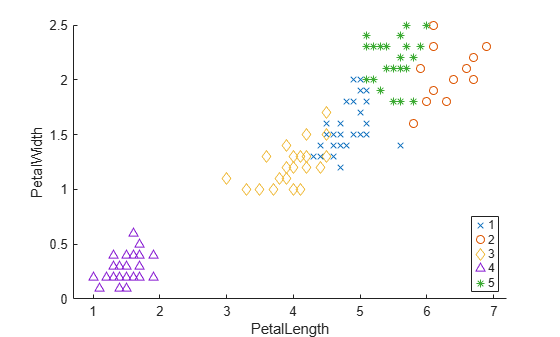# GapEvaluation

Gap criterion clustering evaluation object

## Description

`GapEvaluation` is an object consisting of sample data (`X`), clustering data (`OptimalY`), and gap criterion values (`CriterionValues`) used to evaluate the optimal number of clusters (`OptimalK`). The gap criterion values correspond to the difference , where W is the within-cluster dispersion, `ExpectedLogW` is determined by Monte Carlo sampling from a reference distribution, and `LogW` is computed from the sample data. The optimal number of clusters corresponds to the solution with the largest local or global gap value within a tolerance range (`SearchMethod`). For more information, see Gap Value.

## Creation

Create a gap criterion clustering evaluation object by using the `evalclusters` function and specifying the criterion as `"gap"`.

You can then use `compact` to create a compact version of the gap criterion clustering evaluation object. The function removes the contents of the properties `X`, `OptimalY`, and `Missing`.

## Properties

expand all

### Clustering Evaluation Properties

Clustering algorithm used to cluster the sample data, returned as `'kmeans'`, `'linkage'`, `'gmdistribution'`, or a function handle.

ValueDescription
`'kmeans'`Cluster the data in `X` using the `kmeans` clustering algorithm, with `EmptyAction` set to `"singleton"` and `Replicates` set to `5`.
`'linkage'`Cluster the data in `X` using the `clusterdata` agglomerative clustering algorithm, with `Linkage` set to `"ward"`.
`'gmdistribution'`Cluster the data in `X` using the `gmdistribution` Gaussian mixture distribution algorithm, with `SharedCov` set to `true` and `Replicates` set to `5`.

Data Types: `char` | `function_handle`

Name of the criterion used for clustering evaluation, returned as `'Gap'`.

Criterion values, returned as a numeric vector. Each value corresponds to a proposed number of clusters in `InspectedK`.

Data Types: `double`

Distance metric used for clustering data and computing the criterion values, returned as one of the values in this table or a function handle.

ValueDescription
`'sqEuclidean'`Squared Euclidean distance
`'Euclidean'`Euclidean distance
`'cityblock'`Sum of absolute differences
`'cosine'`One minus the cosine of the included angle between points (treated as vectors)
`'correlation'`One minus the sample correlation between points (treated as sequences of values)

Data Types: `char` | `function_handle`

List of the number of proposed clusters for which to compute criterion values, returned as a positive integer vector.

Data Types: `double`

Optimal number of clusters, returned as a positive integer scalar.

Data Types: `double`

Optimal clustering solution corresponding to `OptimalK`, returned as a positive integer column vector. Each row of `OptimalY` represents the cluster index of the corresponding observation (or row) in `X`. If you specify the clustering solutions as an input argument to `evalclusters` when you create the clustering evaluation object, or if the clustering evaluation object is compact (see `compact`), then `OptimalY` is empty.

Data Types: `double`

Method for selecting the optimal number of clusters, returned as `'globalMaxSE'` or `'firstMaxSE'`.

ValueDescription
`'globalMaxSE'`

Evaluate each proposed number of clusters in `InspectedK` and select the smallest number of clusters satisfying

`$\text{Gap}\left(K\right)\ge GAPMAX-\text{SE}\left(GAPMAX\right),$`

where K is the number of clusters, Gap(K) is the gap value for the clustering solution with K clusters, GAPMAX is the largest gap value, and SE(GAPMAX) is the standard error corresponding to the largest gap value.

`'firstMaxSE'`

Evaluate each proposed number of clusters in `InspectedK` and select the smallest number of clusters satisfying

`$\text{Gap}\left(K\right)\ge \text{Gap}\left(K+1\right)-\text{SE}\left(K+1\right),$`

where K is the number of clusters, Gap(K) is the gap value for the clustering solution with K clusters, and SE(K + 1) is the standard error of the clustering solution with K + 1 clusters.

### Sample Data Properties

Natural logarithm of the within-cluster dispersion W based on the sample data `X`, returned as a numeric vector. W is the within-cluster dispersion computed using the distance metric `Distance`. Each element of `LogW` corresponds to a specific number of proposed clusters (an element of `InspectedK`).

Data Types: `double`

Excluded data, returned as a logical column vector. If an element of `Missing` is `true`, then the corresponding observation (or row) in the data matrix `X` is not used in the clustering solutions. If the clustering evaluation object is compact (see `compact`), then `Missing` is empty.

Data Types: `double` | `logical`

Number of observations in the data matrix `X`, ignoring observations with missing (`NaN`) values, returned as a positive integer scalar.

Data Types: `double`

Data used for clustering, returned as a numeric matrix. Rows correspond to observations, and columns correspond to variables. If the clustering evaluation object is compact (see `compact`), then `X` is empty.

Data Types: `single` | `double`

### Reference Data Properties

Number of reference data sets generated from the reference distribution `ReferenceDistribution`, returned as a positive integer scalar.

Data Types: `double`

Expectation of the natural logarithm of the within-cluster dispersion W based on the generated reference data, returned as a numeric vector. W is the within-cluster dispersion computed using the distance metric `Distance`. Each element of `ExpectedLogW` corresponds to a specific number of proposed clusters (an element of `InspectedK`).

Data Types: `double`

Reference data generation method, returned as `'PCA'` or `'uniform'`.

ValueDescription
`'PCA'`Generate reference data from a uniform distribution over a box aligned with the principal components of the data matrix `X`.
`'uniform'`Generate reference data uniformly over the range of each feature in the data matrix `X`.

Standard error of the natural logarithm of the within-cluster dispersion W with respect to the reference data, returned as a numeric vector. W is the within-cluster dispersion computed using the distance metric `Distance`. Each element of `SE` corresponds to a specific number of proposed clusters (an element of `InspectedK`).

Data Types: `double`

Standard deviation of the natural logarithm of the within-cluster dispersion W with respect to the reference data, returned as a numeric vector. W is the within-cluster dispersion computed using the distance metric `Distance`. Each element of `StdLogW` corresponds to a specific number of proposed clusters (an element of `InspectedK`).

Data Types: `double`

## Object Functions

 `addK` Evaluate additional numbers of clusters `compact` Compact clustering evaluation object `increaseB` Increase reference data sets `plot` Plot clustering evaluation object criterion values

## Examples

collapse all

Evaluate the optimal number of clusters using the gap clustering evaluation criterion.

Load the `fisheriris` data set. The data contains length and width measurements from the sepals and petals of three species of iris flowers.

`load fisheriris`

Evaluate the optimal number of clusters based on the gap criterion values. Cluster the data using `kmeans`.

```rng("default") % For reproducibility evaluation = evalclusters(meas,"kmeans","gap","KList",1:6)```
```evaluation = GapEvaluation with properties: NumObservations: 150 InspectedK: [1 2 3 4 5 6] CriterionValues: [0.0720 0.5928 0.8762 1.0114 1.0534 1.0720] OptimalK: 5 ```

The `OptimalK` value indicates that, based on the gap criterion, the optimal number of clusters is five.

Plot the gap criterion values for each number of clusters tested.

`plot(evaluation)`Based on the plot, the maximum value of the gap criterion occurs at six clusters. However, the value at five clusters is within one standard error of the maximum, so the suggested optimal number of clusters is five.

Create a grouped scatter plot to examine the relationship between petal length and width. Group the data by the suggested clusters.

```PetalLength = meas(:,3); PetalWidth = meas(:,4); clusters = evaluation.OptimalY; gscatter(PetalLength,PetalWidth,clusters,[],"xod^*");```The plot shows cluster 4 in the lower-left corner, completely separated from the other four clusters. Cluster 4 contains flowers with the smallest petal widths and lengths. Cluster 2 is in the upper-right corner, and contains flowers with the largest petal widths and lengths. Cluster 5 is next to cluster 2, and contains flowers with similar petal widths but smaller petal lengths compared to the flowers in cluster 2. Clusters 1 and 3 are near the center of the plot, and contain flowers with measurements between the extremes.